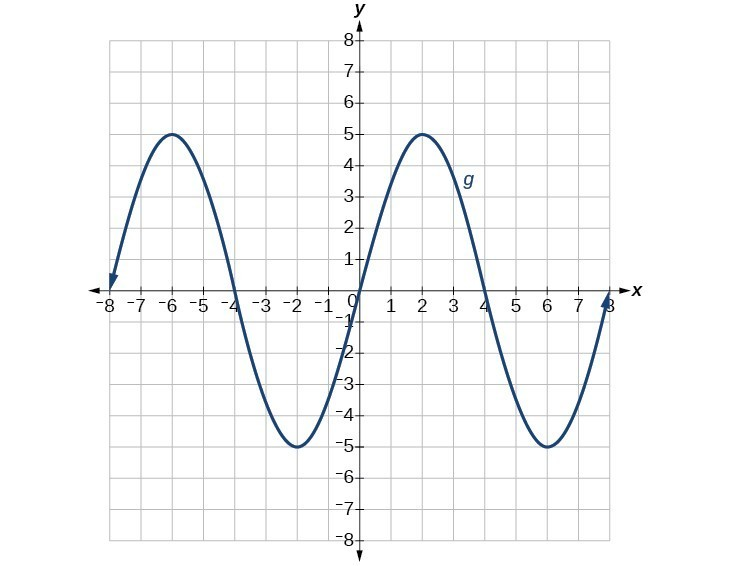Solutions to Try Its

1. The graphs of $f\left(x\right)$ and $g\left(x\right)$ are shown below. The transformation is a horizontal shift. The function is shifted to the left by 2 units.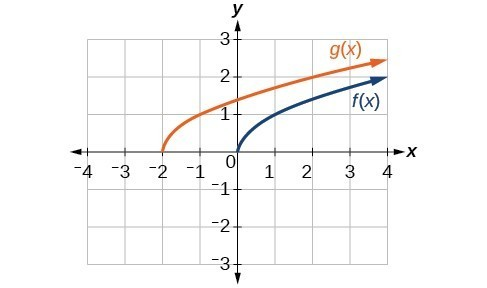2.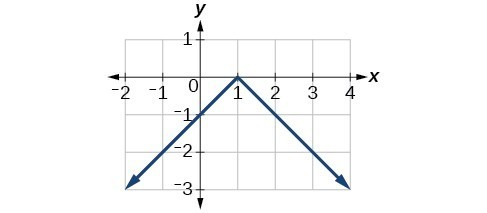a)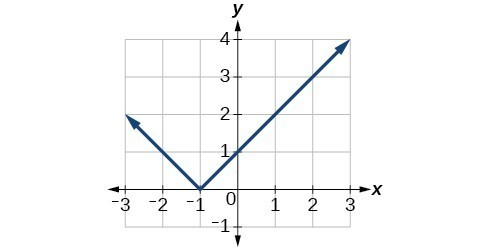b)

3. $g\left(x\right)=-f\left(x\right)$

 $x$ -2 0 2 4 $g\left(x\right)$ $-5$ $-10$ $-15$ $-20$

$h\left(x\right)=f\left(-x\right)$

 $x$ -2 0 2 4 $h\left(x\right)$ 15 10 5 unknown

4. even

5.

 $x$ 2 4 6 8 $g\left(x\right)$ 9 12 15 0

6. $g\left(x\right)=3x - 2$

7. $g\left(x\right)=f\left(\frac{1}{3}x\right)$ so using the square root function we get $g\left(x\right)=\sqrt{\frac{1}{3}x}$

8.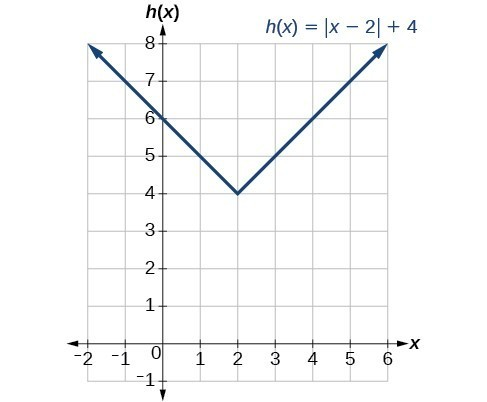9. $g\left(x\right)=\frac{1}{x - 1}+1$

10. Notice: $g\left(x\right)=f\left(-x\right)$ looks the same as $f\left(x\right)$ .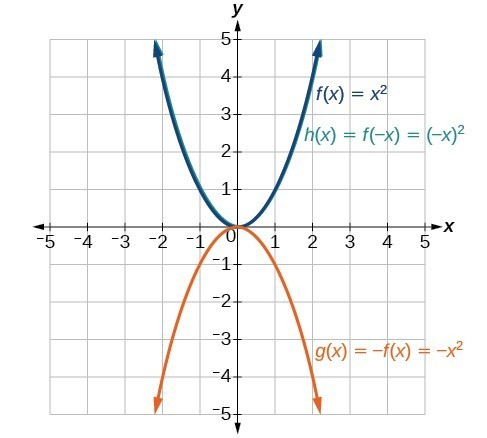Solution to Odd-Numbered Exercises

1. A horizontal shift results when a constant is added to or subtracted from the input. A vertical shifts results when a constant is added to or subtracted from the output.

3. A horizontal compression results when a constant greater than 1 is multiplied by the input. A vertical compression results when a constant between 0 and 1 is multiplied by the output.

5. For a function $f$, substitute $\left(-x\right)$ for $\left(x\right)$ in $f\left(x\right)$. Simplify. If the resulting function is the same as the original function, $f\left(-x\right)=f\left(x\right)$, then the function is even. If the resulting function is the opposite of the original function, $f\left(-x\right)=-f\left(x\right)$, then the original function is odd. If the function is not the same or the opposite, then the function is neither odd nor even.

7. $g\left(x\right)=|x - 1|-3$

9. $g\left(x\right)=\frac{1}{{\left(x+4\right)}^{2}}+2$

11. The graph of $f\left(x+43\right)$ is a horizontal shift to the left 43 units of the graph of $f$.

13. The graph of $f\left(x - 4\right)$ is a horizontal shift to the right 4 units of the graph of $f$.

15. The graph of $f\left(x\right)+8$ is a vertical shift up 8 units of the graph of $f$.

17. The graph of $f\left(x\right)-7$ is a vertical shift down 7 units of the graph of $f$.

19. The graph of $f\left(x+4\right)-1$ is a horizontal shift to the left 4 units and a vertical shift down 1 unit of the graph of $f$.

21. decreasing on $\left(-\infty ,-3\right)$ and increasing on $\left(-3,\infty \right)$

23. decreasing on $\left(0,\infty \right)$

25.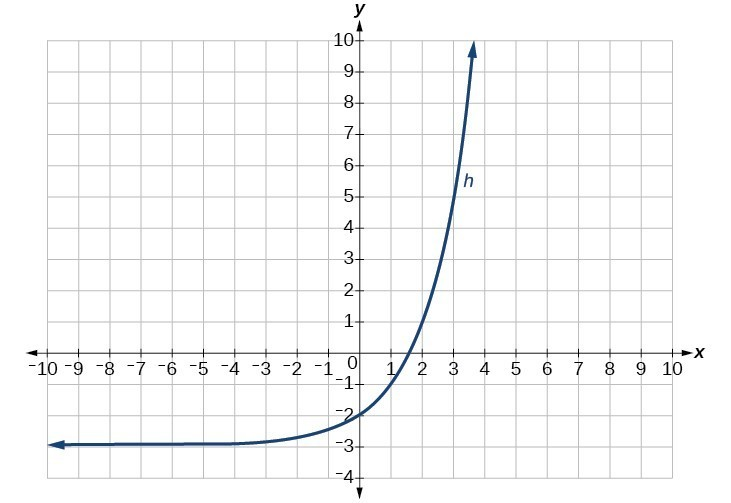27.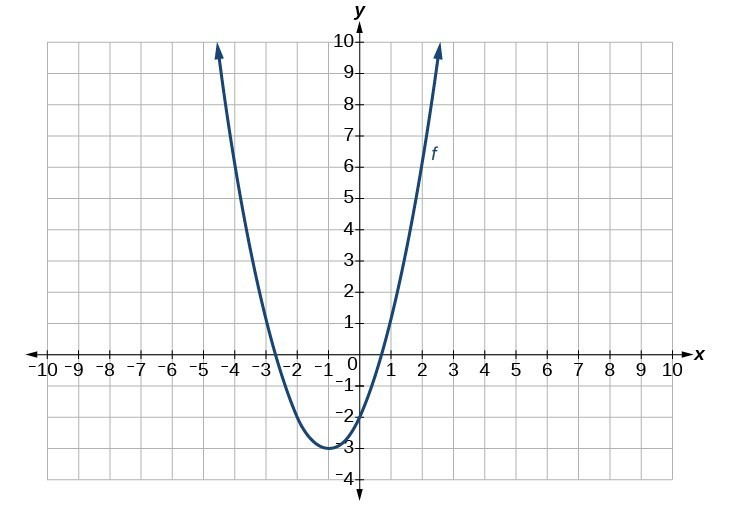29.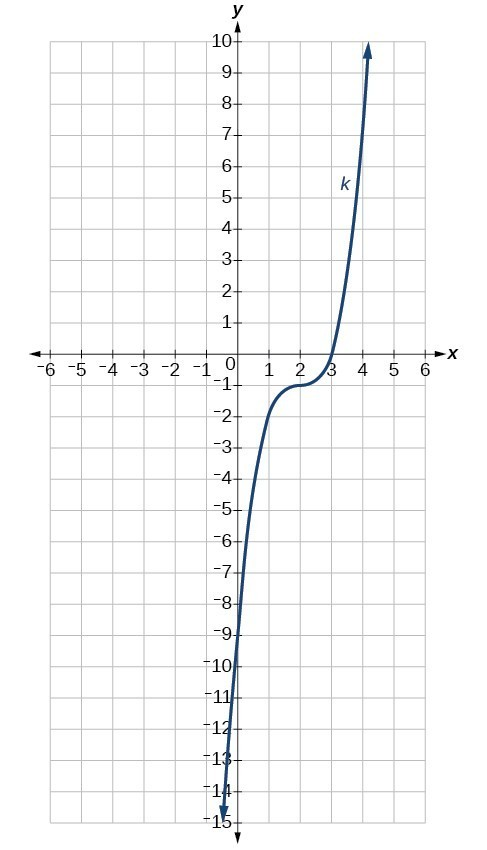31. $g\left(x\right)=f\left(x - 1\right),h\left(x\right)=f\left(x\right)+1$

33. $f\left(x\right)=|x - 3|-2$

35. $f\left(x\right)=\sqrt{x+3}-1$

37. $f\left(x\right)={\left(x - 2\right)}^{2}$

39. $f\left(x\right)=|x+3|-2$

41. $f\left(x\right)=-\sqrt{x}$

43. $f\left(x\right)=-{\left(x+1\right)}^{2}+2$

45. $f\left(x\right)=\sqrt{-x}+1$

47. even

49. odd

51. even

53. The graph of $g$ is a vertical reflection (across the $x$ -axis) of the graph of $f$.

55. The graph of $g$ is a vertical stretch by a factor of 4 of the graph of $f$.

57. The graph of $g$ is a horizontal compression by a factor of $\frac{1}{5}$ of the graph of $f$.

59. The graph of $g$ is a horizontal stretch by a factor of 3 of the graph of $f$.

61. The graph of $g$ is a horizontal reflection across the $y$ -axis and a vertical stretch by a factor of 3 of the graph of $f$.

63. $g\left(x\right)=|-4x|$

65. $g\left(x\right)=\frac{1}{3{\left(x+2\right)}^{2}}-3$

67. $g\left(x\right)=\frac{1}{2}{\left(x - 5\right)}^{2}+1$

69. The graph of the function $f\left(x\right)={x}^{2}$ is shifted to the left 1 unit, stretched vertically by a factor of 4, and shifted down 5 units.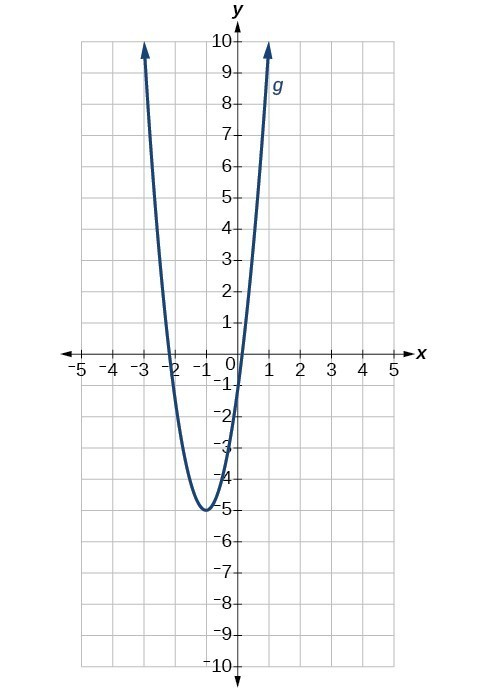71. The graph of $f\left(x\right)=|x|$ is stretched vertically by a factor of 2, shifted horizontally 4 units to the right, reflected across the horizontal axis, and then shifted vertically 3 units up.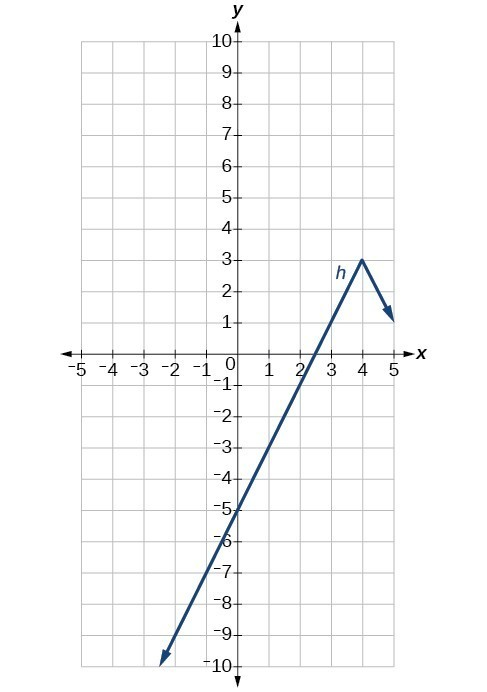73. The graph of the function $f\left(x\right)={x}^{3}$ is compressed vertically by a factor of $\frac{1}{2}$.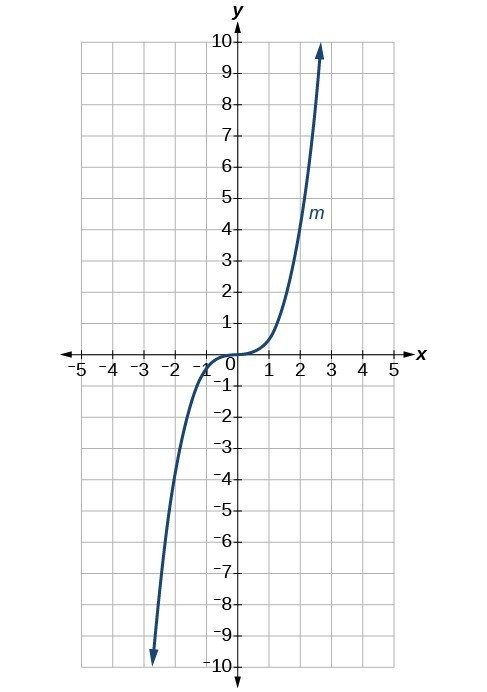75. The graph of the function is stretched horizontally by a factor of 3 and then shifted vertically downward by 3 units.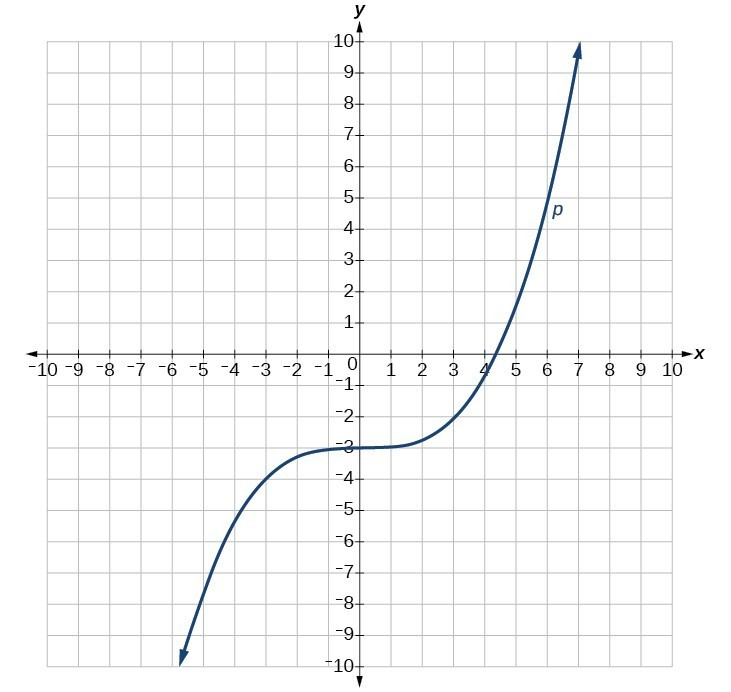77. The graph of $f\left(x\right)=\sqrt{x}$ is shifted right 4 units and then reflected across the vertical line $x=4$.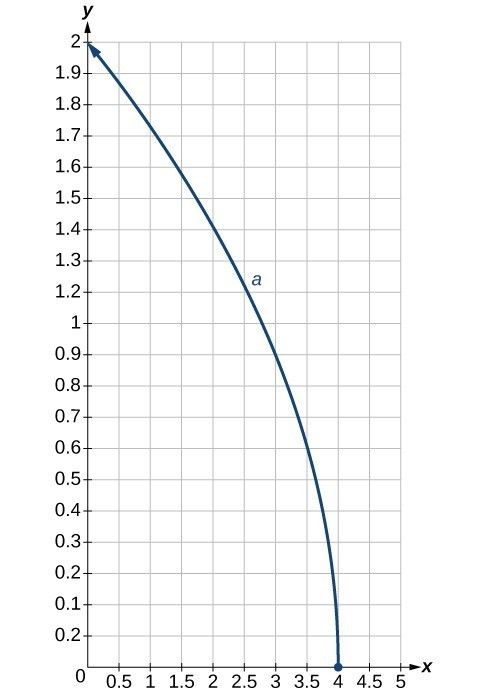79.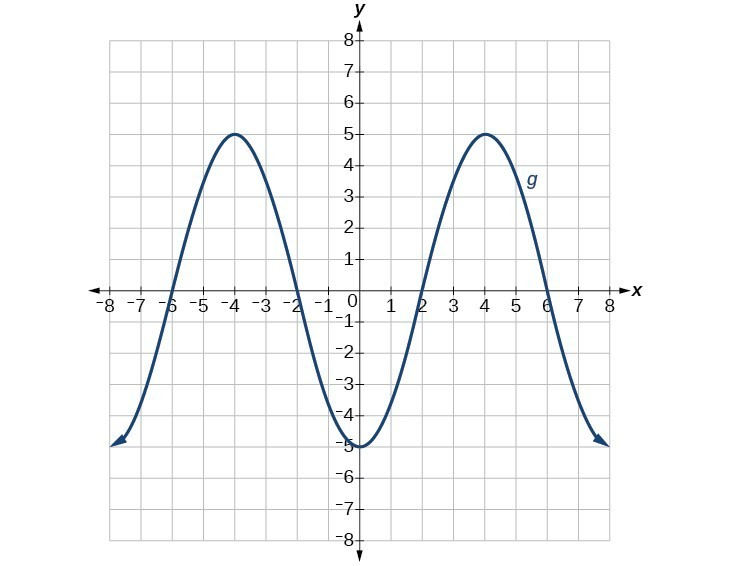81.